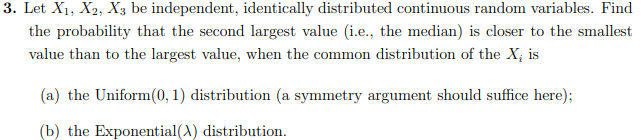Home / Answered Questions / Other / 3-let-x1-x2-x3-be-independent-identically-distributed-continuous-random-variables-find-the-probabili-aw689

# (Solved): 3. Let X1, X2, X3 Be Independent, Identically Distributed Continuous Random Variables. Find The Prob...3. Let X1, X2, X3 be independent, identically distributed continuous random variables. Find the probability that the second largest value i.e., the median) is closer to the smallest value than to the largest value, when the common distribution of the X, is (a) the Uniform(0,1) distribution (a symmetry argument should suffice here); (b) the Exponential(a) distribution.

We have an Answer from Expert